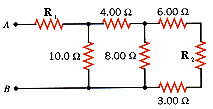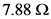# Find the equivalent resistance between points A and B in the drawing (R1 = 3.00 Ω, R2 = 9.00 Ω)

Find the equivalent resistance between points A and B in the drawing (R1 = 3.00 Ω, R2 = 9.00 Ω).The equivalent resistance between points A and B is.